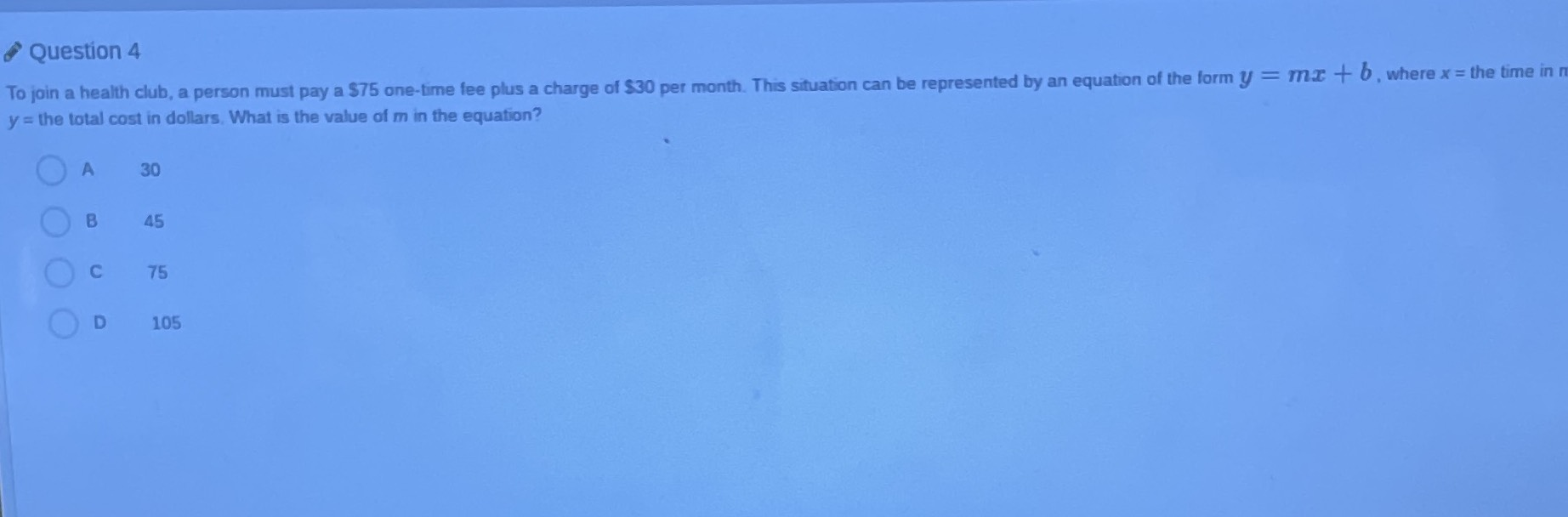### Still have math questions?

Algebra
QuestionTo join a health club, a person must pay a $$\ 75$$ one-time fee plus a charge of $$\ 30$$ per month. This situation can be represented by an equation of the form $$y = m x + b$$ , where $$x =$$ the time and$$y =$$ the total cost in dollars. What is the value of $$m$$ in the equation?

A. 30

B. 45

C. 75

D. 105